# RMS Voltage: What it is? (Formula And How To Calculate It)

Contents

## What is RMS Voltage?

The word RMS stands for Root Mean Square. An RMS voltage is defined as the square root of the mean square of instantaneous values of the voltage signal. The RMS is also known as the quadratic mean. RMS voltage can also be defined for a continuously varying voltage in terms of an integral of the squares of the instantaneous values during a cycle.

The RMS value is most important in the case of an AC signal. Because the instantaneous value of an AC signal varies continuously with respect to time. Unlike a DC signal, which is relatively constant.

Therefore, the instantaneous value of voltage cannot directly be used for the calculation.

The RMS voltage is also known as the equivalent DC voltage because the RMS value gives the amount of AC power drawn by a resistor similar to the power drawn by a DC source.

For example, take a 5Ω load connected with a 10V DC source. In the case of the DC source, the value of voltage is constant for every instant of time. Hence, the power drawn by the load is easily calculated, and it is 20W.

But instead of a DC source, say we use an AC source. In this condition, the value of voltage is varying with respect to time, as shown in the figure below.

The AC signal is a sinusoidal wave signal in most conditions, as shown in the above figure. Since in a sinusoidal wave signal the instantaneous value varies, we cannot use the instantaneous value to calculate the power.

But if we find the RMS value of the above signal, we can use it to find the power. Let say the RMS value is 10Vrms. The power dissipates by the load is 20W.

The voltage we received at home is RMS voltage. Multimeters also give an RMS value for AC power. And in a power system, we use system voltage that is also an RMS value.

## How to Calculate RMS Voltage

The RMS value is only calculated for the time-varying waveforms where the magnitude of quantity varies with respect to time.

We cannot find the RMS value for the DC waveform as the DC waveform has a constant value for every instant of time.

There are two methods to calculate RMS value.

• Graphical Method
• Analytical Method

### Graphical Method

In this method, we use a waveform to find the RMS value. The graphical method is more useful when the signal is not symmetrical or sinusoidal.

The accuracy of this method depends on the number of points taken from the waveform. Few points result in low accuracy, and a more significant number of points result in high accuracy.

The RMS value is a square root of the average value of the squared function. For example, let’s take a sinusoidal waveform of voltage as shown below figure.

Follow these steps to calculate the RMS voltage by graphical method.

Step-1: Divide waveform into equal parts. Here, we consider the half cycle of the waveform. You can consider full-cycle also.

The first half cycle divides into ten equal parts; V1, V2, …, V10.

Step-2: Find square of each value.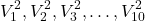Step-3: Take the average of these squared values. Find the total of these values and divide by the total number of points.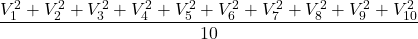Step-4 Now, take square root of this value.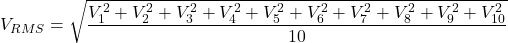These steps are same for all type of continuous waveforms.

For different types of time-varying signals like triangular, square; these steps follow to find the RMS voltage.

Let’s solve these steps with an example.

Find the RMS value of the waveform shown in the figure below. Consider a pure sinusoidal wave of voltage.

Step-1: First half-cycle divides into ten equal parts. And the values of these parts are as shown in the figure.

Step-2: Find a Square of each point.

Step-3: Take average of squared values.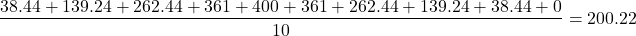Step-4: Find square root.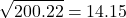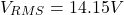### Analytical Method

In this method, the RMS voltage can be calculated by a mathematical procedure. This method is more accurate for the pure sinusoidal waveform.

Consider a pure sinusoidal voltage waveform defined as VmCos(ωt) with a period of T.

Where,

Vm = Maximum value or Peak value of voltage waveform

ω = Angular frequency = 2π/T

Now, we calculate the RMS value of voltage.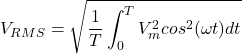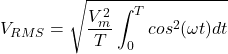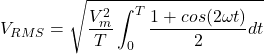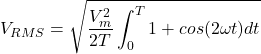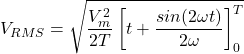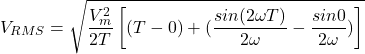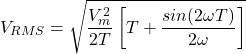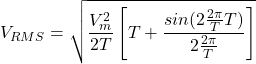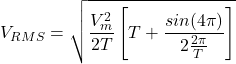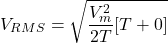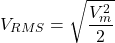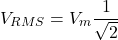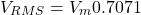So, RMS value of pure sinusoidal waveform can derive from the peak (maximum) value.

In above example (graphical method), the peak value is 20V.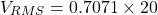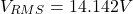## RMS Voltage Formula

RMS voltage can be calculated from peak value, peak-to-peak value, and average value.

For sinusoidal waveform below formulas are used to calculate the RMS voltage.

From peak voltage (VP);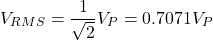From peak to peak voltage (VPP);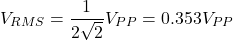From average voltage (VAVG);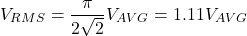## RMS Voltage vs Peak Voltage vs Peak-to-Peak Voltage vs Average Voltage

The RMS voltage is essential for various calculations in AC circuits. Similarly, peak voltage, peak-to-peak voltage, and average voltage are also necessary.

### Peak Voltage

Peak voltage is defined as the maximum value of voltage for any voltage waveform. The peak value measures from the reference axis (0) to the highest point of the waveform.

If we consider a sinusoidal waveform, the value of voltage increases from the reference axis and reaches the peak point of the waveform on the positive side. The difference between these two points gives us the positive peak voltage.

From the peak point, the voltage starts decreasing and reaches to reference axis. After that, it starts increasing on the negative side and reaches the peak point. This point is a negative peak point.

We can calculate peak voltage from the RMS voltage, peak-to-peak voltage, and average voltage.

#### Peak Voltage From RMS Voltage

To calculate the peak voltage from RMS voltage, we need to multiply RMS voltage by an approximate factor of 1.414.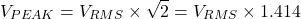#### Peak Voltage From Peak-to-Peak Voltage

The peak voltage is half of the peak-to-peak voltage.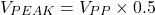#### Peak Voltage From Average Voltage

To calculate the peak voltage from average voltage, we need to multiply the average voltage by an approximate factor of 1.57.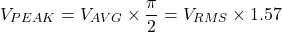### Peak-to-Peak Voltage

Peak-to-peak voltage is a difference between positive peak voltage and negative peak voltage.

For a sinusoidal waveform, peak-to-peak voltage is shown below figure.

We can calculate Peak-to-peak voltage from the RMS voltage, peak voltage, and average voltage.

#### Peak-to-Peak Voltage From RMS Voltage

To calculate peak-to-peak voltage from RMS voltage, 2.8284 is the approximate multiplier factor.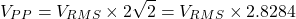#### Peak-to-Peak Voltage From Peak Voltage

Peak-to-peak voltage is twice of the peak voltage.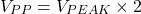#### Peak-to-Peak Voltage From Average Voltage

To calculate peak-to-peak voltage from RMS voltage, 3.14 (π) is the approximate multiplier factor.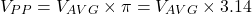### Average Voltage

The method to find the average voltage is similar to the RMS voltage. The only difference is that the instantaneous values are not squared function and do not make square root.

The average value gives us the horizontal line. And the area above the horizontal line is the same as the area below the horizontal line. It is also known as means voltage.

We can calculate the average voltage from the RMS voltage, peak voltage, and peak-to-peak voltage.

#### Average Voltage From RMS Voltage

To calculate the average voltage from RMS voltage, 0.9 is the approximate multiplier factor.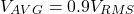#### Average Voltage From Peak Voltage

To calculate the average voltage from peak voltage, 0.637 is the approximate multiplier factor.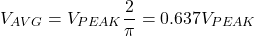#### Average Voltage From Peak-to-Peak Voltage

To calculate the average voltage from peak-to-peak voltage, 0.318 is the approximate multiplier factor.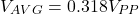Want To Learn Faster? 🎓
Get electrical articles delivered to your inbox every week.
No credit card required—it’s 100% free.